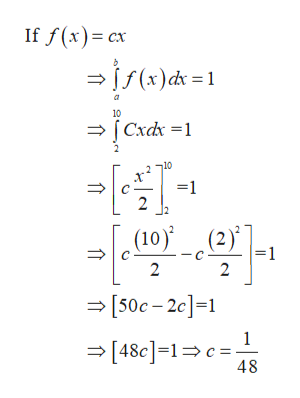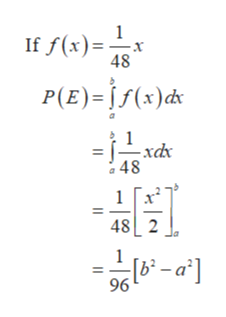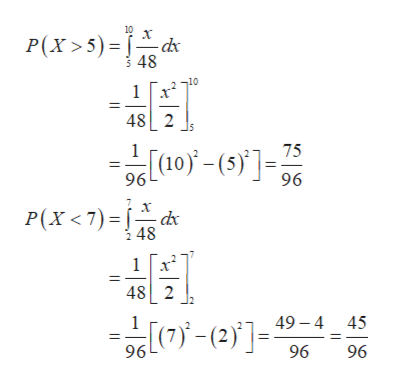# Suppose you choose a real number X from the interval [2, 10] with a density function of the form f(x) = Cx, where C is a constant.(a) Find C.(b) Find P(E), where E = [a, b] is a subinterval of [2, 10].(c) Find P(X > 5), P(X < 7), and P(X2 − 12X + 35 > 0).

Question

Suppose you choose a real number X from the interval [2, 10] with a density function of the form f(x) = Cx, where C is a constant.
(a) Find C.
(b) Find P(E), where E = [a, b] is a subinterval of [2, 10].
(c) Find P(X > 5), P(X < 7), and P(X2 − 12X + 35 > 0).

check_circleExpert Solution
Step 1

Part (a)

Here the given function is f(x) = C x

Where C is a constant.

Find the constant function C and the given interval is [2, 10]help_outlineImage TranscriptioncloseIf f(x) cx - js(x)ck =1 a 10 Cxdx 1 10 1 2 (10)(2) (2)) -1 2 2 - [50c-2c]=1 1 [48e]-1c= 48 fullscreen
Step 2

Part (b)

Find P(E), where E = [a, b] is a subinterval of [2, 10].help_outlineImage TranscriptioncloseIf f(x)=1 x 48 P(E)= f(x)ds 1 a48 1 48 2 - a] 96 fullscreen
Step 3

Part (c):

P(E), where E = [a, b] is a subinterval of [2, 10].
Now Find P(X > 5), P(X <...help_outlineImage Transcriptionclose10 -dx P(x>5) 48 10 1 48 2 1 75 96 96 P(x 7) 48 1 48 2 49-4 45 (2 96 96 96 fullscreen

### Want to see the full answer?

See Solution

#### Want to see this answer and more?

Solutions are written by subject experts who are available 24/7. Questions are typically answered within 1 hour*

See Solution
*Response times may vary by subject and question
Tagged in

### Probability